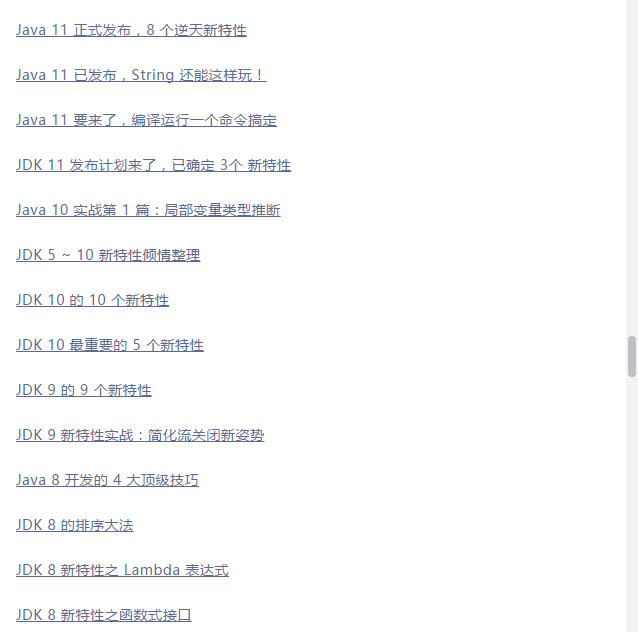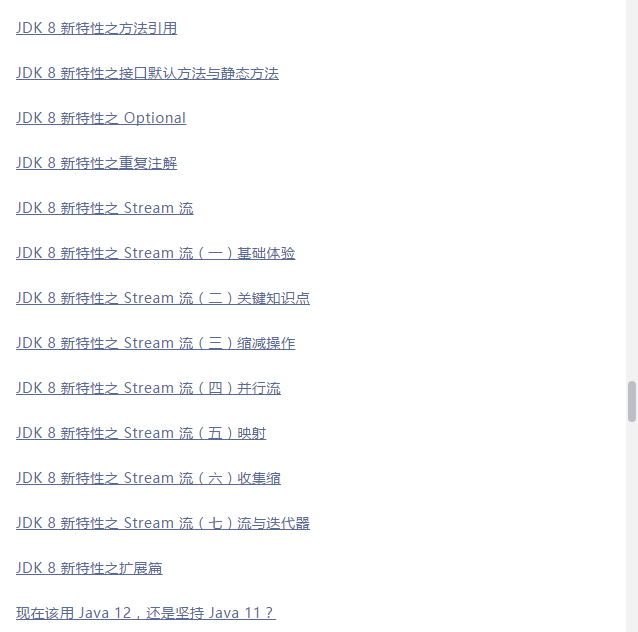Java 13 都快要来了，12必须跟栈长学起！

Java 13 即将发布，新特性必须抢先看！

Java 12 已经发布数月了：Java 12 正式发布，8大新特性！，今天我再来分享下在 Java 12 中关于 String 的三个骚操作，绝逼有用。

## 1、transform

transform：即字符串转换，来看下 transform 的实现源码：

public <R> R transform(Function<? super String, ? extends R> f) {
return f.apply(this);
}


private static void testTransform() {
System.out.println("======test java 12 transform======");
List list1 = List.of("Java", " Python", " C++ ");
List list2 = new ArrayList<>();

list1.forEach(element ->
.transform(String::toUpperCase)
.transform((e) -> "Hi," + e))
);

list2.forEach(System.out::println);
}


======test java 12 transform======
Hi,JAVA
Hi,PYTHON
Hi,C++


## 2、indent

private static void testIndent() {
System.out.println("======test java 12 indent======");
String result = "Java\n Python\nC++".indent(3);
System.out.println(result);
}


======test java 12 indent======
Java
Python
C++


private String indent(int n, boolean removeBlanks) {
Stream<String> stream = removeBlanks ? lines(Integer.MAX_VALUE, Integer.MAX_VALUE)
: lines();
if (n > 0) {
final String spaces = " ".repeat(n);
stream = stream.map(s -> spaces + s);
} else if (n == Integer.MIN_VALUE) {
} else if (n < 0) {
stream = stream.map(s -> s.substring(Math.min(-n, s.indexOfNonWhitespace())));
}
return stream.collect(Collectors.joining("\n", "", "\n"));
}


## 3、describeConstable

private static void testDescribeConstable() {
System.out.println("======test java 12 describeConstable======");
String name = "Java技术栈";
Optional optional = name.describeConstable();
System.out.println(optional.get());
}


======test java 12 describeConstable======
Java技术栈


Java 12, String 实现了 Constable 接口：

java.lang.constant.Constable

public interface Constable {

Optional<? extends ConstantDesc> describeConstable();

}


Java 12 String 的实现源码：

@Override
public Optional<String> describeConstable() {
return Optional.of(this);
}Java 12 新特性继续更新中……